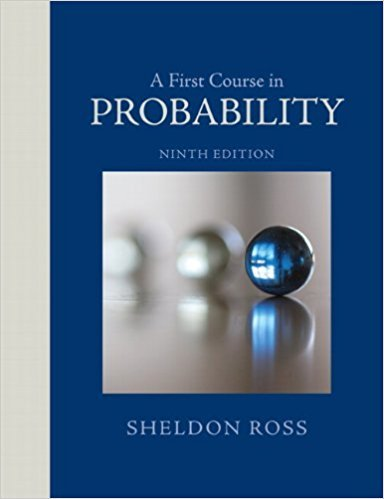×
Get Full Access to A First Course In Probability - 9 Edition - Chapter 4 - Problem 12p
Get Full Access to A First Course In Probability - 9 Edition - Chapter 4 - Problem 12p

×

# In the game of Two-Finger Morra, 2 players show 1 or 2ISBN: 9780321794772 63

## Solution for problem 12P Chapter 4

A First Course in Probability | 9th Edition

• Textbook Solutions
• 2901 Step-by-step solutions solved by professors and subject experts
• Get 24/7 help from StudySoup virtual teaching assistantsA First Course in Probability | 9th Edition

4 5 1 364 Reviews
26
5
Problem 12P

Problem 12P

In the game of Two-Finger Morra, 2 players show 1 or 2 fingers and simultaneously guess the number of fingers their opponent will show. If only one of the players guesses correctly, he wins an amount (in dollars) equal to the sum of the fingers shown by him and his opponent. If both players guess correctly or if neither guesses correctly, then no money is exchanged. Consider a specified player, and denote by X the amount of money he wins in a single game of Two-Finger Morra.

(a) If each player acts independently of the other, and if each player makes his choice of the number of fingers he will hold up and the number he will guess that his opponent will hold up in such a way that each of the 4 possibilities is equally likely, what arc the possible values of A and what are their associated probabilities?

(b) Suppose that each player acts independently of the other. If each player decides to hold up the same number of fingers that he guesses his opponent will hold up, and if each player is equally likely to hold up 1 or 2 fingers, what are the possible values of X and their associated probabilities?

Step-by-Step Solution:

Solution:

Step 1 of 3:

It is given that in a Two-finger Morra game, the two players shows 1 or 2 fingers and simultaneously guess the number of fingers showed by the opponent.

Idf both the players failed to guess the number, they both won’t get any amount .If one of the player guesses correctly, then he will get a sum of the amount equal to the number of fingers showed by the two players.

Also, it is given that X denotes the amount of money owned by a player in a Two-Finger Morra game.

Using this we need to find the required values.

Step 2 of 3

Step 3 of 3

##### ISBN: 9780321794772

Since the solution to 12P from 4 chapter was answered, more than 809 students have viewed the full step-by-step answer. The answer to “In the game of Two-Finger Morra, 2 players show 1 or 2 fingers and simultaneously guess the number of fingers their opponent will show. If only one of the players guesses correctly, he wins an amount (in dollars) equal to the sum of the fingers shown by him and his opponent. If both players guess correctly or if neither guesses correctly, then no money is exchanged. Consider a specified player, and denote by X the amount of money he wins in a single game of Two-Finger Morra.(a) If each player acts independently of the other, and if each player makes his choice of the number of fingers he will hold up and the number he will guess that his opponent will hold up in such a way that each of the 4 possibilities is equally likely, what arc the possible values of A and what are their associated probabilities?(b) Suppose that each player acts independently of the other. If each player decides to hold up the same number of fingers that he guesses his opponent will hold up, and if each player is equally likely to hold up 1 or 2 fingers, what are the possible values of X and their associated probabilities?” is broken down into a number of easy to follow steps, and 203 words. This full solution covers the following key subjects: fingers, player, hold, opponent, guess. This expansive textbook survival guide covers 4 chapters, and 469 solutions. A First Course in Probability was written by and is associated to the ISBN: 9780321794772. This textbook survival guide was created for the textbook: A First Course in Probability , edition: 9. The full step-by-step solution to problem: 12P from chapter: 4 was answered by , our top Statistics solution expert on 08/11/17, 08:57AM.

Unlock Textbook Solution